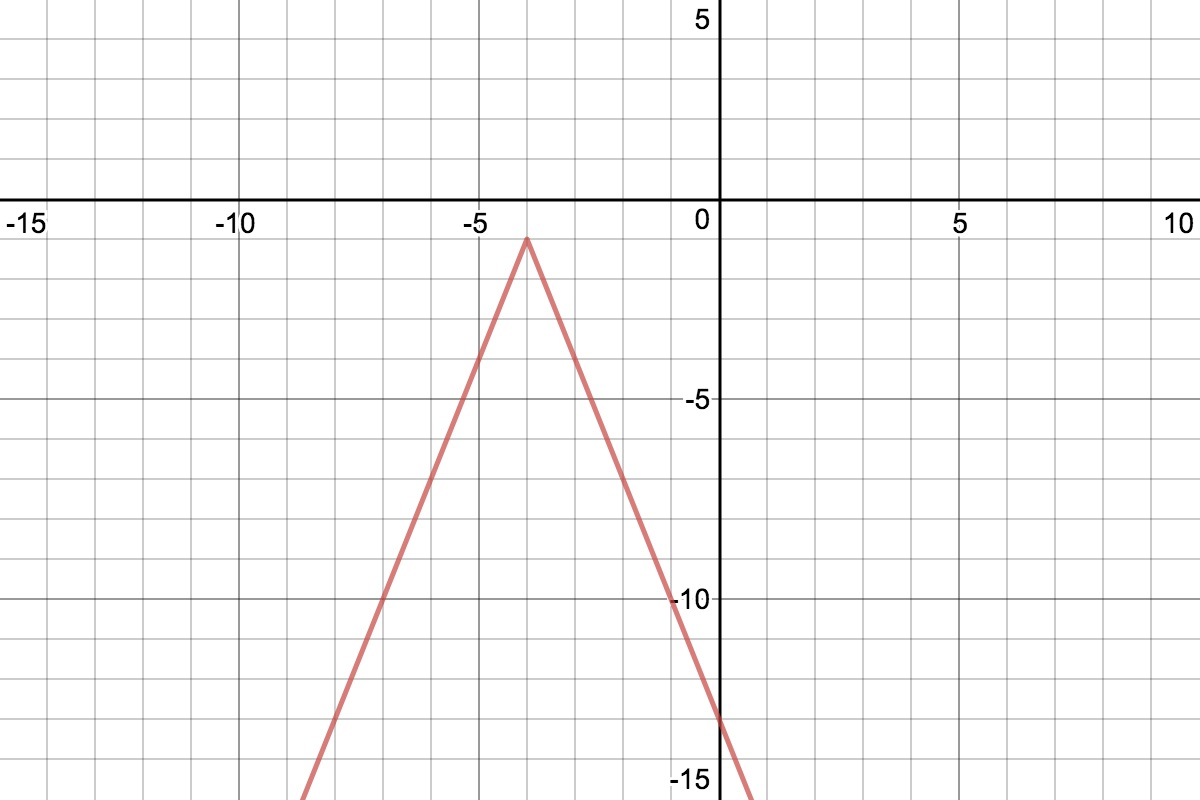# LINR 3 | Lesson 3 | Practice SolutionGraph is created using Desmos.com.

1. Notice that the vertex is at $$(-4, -1)$$; therefore there is a translation to the left 4 and down 1. There is also a vertical stretch of 3 and a reflection over the $$x$$-axis.
2. Write any equation of your choosing, in the form $$y=a|x-h|+k$$, sketch the graph and discuss the translation, the vertical stretch or shrink and any reflection.